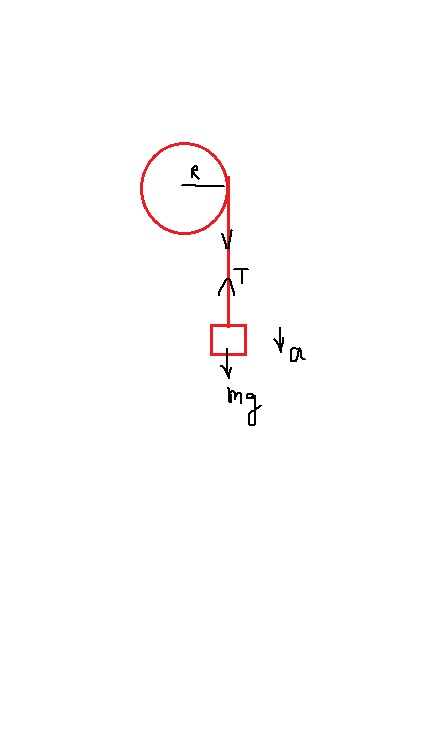# A thin light wire is wrapped around the rim of a wheel. The wheel rotates without friction about...

## Question:

A thin light wire is wrapped around the rim of a wheel. The wheel rotates without friction about a stationary horizontal axis that passes through the center of the wheel. The wheel is a uniform disk with radius R = 0.280 m and I = {eq}\frac{1}{2} M R^{2} {/eq}. An object of mass m = 4.20 kg is suspended from the free end of the wheel. The system is released from rest and the suspended object descends with constant acceleration.

If the suspended object moves downward a distance of 3.00 m in 2.00 s, what is the mass of the wheel?

## Rotational motion

We have to use the concept and equation of the rotational motion to find the solution to this problem. We have to use the rotational kinematics equation to establish the relation between the torque, moment of inertia and angular acceleration.Given

Distance fell by the mass (S) = 3 m

time taken (t) = 2 s

Now using the kinematic equation

{eq}\displaystyle S = ut + 0.5at^{2} \\ 3 = 0 + (0.5a*2^{2}) \\ a = 1.5 \ m/s^{2} {/eq}

Now considering the FBD of mass m

{eq}\displaystyle mg - T = ma \\ T = m(g-a) \\ T = 4.2(9.81-1.5)\\ T = 34.9 \ N {/eq}

Now the angular acceleration of the wheel would be

{eq}\displaystyle \alpha = \dfrac{a}{R} \\ \alpha = \dfrac{1.5}{0.28} \\ \alpha = 5.36 \ rad/s^{2} {/eq}

Now, torque is defined as

{eq}\displaystyle \tau = I \alpha \\ T*R = 0.5MR^{2} \alpha \\ T = 0.5MR \alpha \\ 34.9 = 0.5*M*0.28*5.36 \\ M = 46.53 \ Kg {/eq}